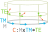## § Connections, take 2

• I asked a math.se question about position, velocity, acceleration that recieved a great answer by peek-a-boo. Let me try and provide an exposition of his answer.
• Imagein a base manifold $M$, say a circle.
• Now imagine a vector bundle over this, say 2D spaces lying above each point on the circle. Call this $(E, \pi, M)$
• What is a connection? Roughly speaking, it seems to be a device to convert elements of $TM$ into elements of $TE$.
• We imagine the base manifold (circle) as horizontal, and the bundle $E$ as vertical. We imagine $TM$ as vectors lying horizontal on the circle, and we imagine $TE$ as vectors lying horizontal above the bundle. So something like:• So the connection has type $C: E \times TM \to TE$. Consider a point $m \in M$ in the base manifold.
• Now think of the fiber $E_m \subseteq E$ over $x$.
• Now think of any point $e \in E_m$ in the fiber of $m$.
• This gives us a map $C_e: T_e M \to T_e E$, which tells us to imagine a particle $e \in E$ following its brother in $m \in M$. If we know the velocity $\dot m \in T_m M$, we can find the velocity of the sibling upstrairs with $C_e(\dot m)$.
• In some sense, this is really like path lifting, except we're performing "velocity lifting". Given a point in the base manifold and a point somewhere upstairs in the cover (fiber), we are told how to "develop" the path upstairs given information about how to "develop" the path downstairs.
• I use "develop" to mean "knowing derivatives".

#### § Differentiating vector fields along a curve

• Given all of this, suppose we have a curve $c: I to M$ and a vector field over the curve $v: I \to E$ such that the vector field lies correctly over the curve; $\pi \circ v = c$. We want to differentiate $v$, such that we get another $v': TI \to E$.
• That's the crucial bit, $v$ and $v'$ have the same type, and this is achieved through the connection. So a vector field and its derivative are both vector fields over the curve.
• How do we do this? We have the tangent mapping $Tv: TI \mapsto TE$.
• We kill off the component given by pushing forward the tangent vector $Tc(i): TI$ at the bundle location $v(i)$ via the connection. This kills of the effect of the curving of the curve when measuring the change in the vector field $v$.
• We build $[z(t_i: TI) \equiv Tv(ti) - C_{v(i)}(Tc(i))]: TI \to TE$.
• We now have a map from $I$ to $TE$, but we want a map to $E$. What do?
• Well, we can check that the vector field we have created is a vertical vector field, which means that it lies entirely within the fiber. Said differently, we check that it pushes forward to the zero vector under projection, so $TM: TE \to TM$ will be zero for the image of $w$.
• This means that $z$ lies entirely "inside" each fiber, or it lies entirely in the tangent to the vector space $\pi^{-1}(m)$ (ie, it lives in $T\pi^{-1}(m)$), instead of living in the full tangent bundle $E_m$ where it has access to the horizontal components.
• But for a vector space, the tangent space is canonically isomorphic to the vector space itself! (parallelogram law/can move vectors around/...). Thus, we can bring down the image of $w$ from $TE$ down to $E$!
• This means we now have a map $z: TI \to E$.
• But we want a $w: I \to E$. See that the place where we needed a $TI$ was to produce